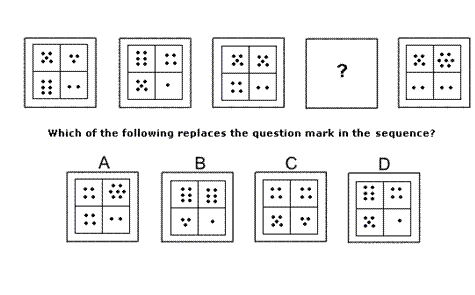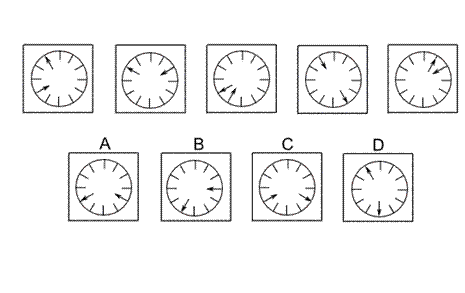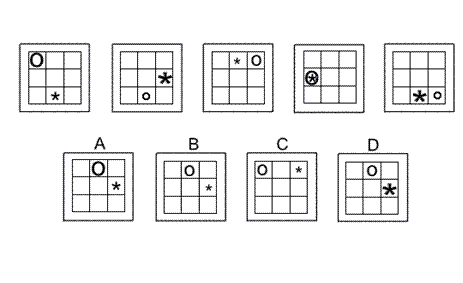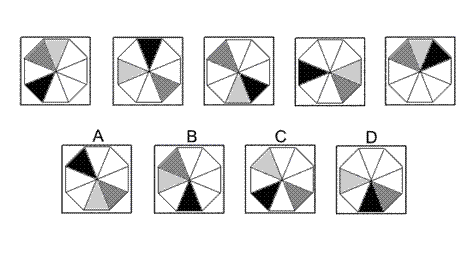Aptitude Tests 4 Me

Non Verbal Logic

Detailed solution

26.Solution: C Explanation: In this question, the pattern of squares and rectangles follows two rules alternately. The first rule is a rotation of 90° anticlockwise. The second rule is a reflection in the vertical axis. Following these rules, to obtain the next diagram of the sequence, the first rule should be applied next and the correct answer could be A, B or C. At the same time, the shading of the non-white tiles changes and follows this sequence: black changes to dark grey, changes to medium grey, changes to light grey, changes to medium grey, changes to dark grey, changes to black etc. Following this rule, the correct answer is C.

27.Solution: B Explanation: In this question there are two rules to follow that are applied alternately. The first rule is that the numbers of dots in the top row increase by one while the numbers of dots in the second row decrease by one. The second rule is that the numbers of dots in the left column decrease by one while the numbers of dots in the right column increase by one. To obtain the missing diagram of the sequence, the first rule should be applied and the correct answer is B.

28.Solution: A Explanation: In this question there is a dial with two hands - one hand pointing towards the centre of the dial, the other pointing away from the centre of the dial. Each hand follows its own rule. The hand pointing towards the centre of the dial moves clockwise by six places, then five places, then four etc. The hand pointing away from the centre of the dial moves anticlockwise by one place, then two places, then three etc. To find the next diagram of the sequence, therefore, the hand pointing towards the centre should move clockwise by two places and the hand pointing away from the centre should move anticlockwise by five places. The correct answer, therefore, is A.

29.Solution: B Explanation: In this question there is an asterisk and a letter O which each follows its own rule. The asterisk moves anticlockwise, each time by 90°, around the four squares along the edges of the grid. The letter O moves two squares in one direction and one square in the other direction, following a letter L-shaped path like the Knight's move in chess, but always going clockwise around the grid. Following these rules, the correct answer could be A, B or D. However the size of the two symbols also changes from small to medium to large, back to small etc. Following this rule, for the next diagram of the sequence, the asterisk should be small and the letter O should be medium. The correct answer, therefore, is B.

30.Solution: D Explanation: In this question there is a black triangle, a medium grey triangle and a light grey triangle each of which follows its own rule. The black triangle rotates around the octagon by 135° clockwise each time. The medium grey triangle rotates by 180° each time. The light grey triangle rotates by 90° anticlockwise each time. Following these rules, the correct answer is D.

 1 2 3 4 5 6 7 8 9 10 11 12 13 14 15 16 17 18 19 20 21 22 23 24 25 26 27 28 29 30 31 32 33 34 35 36 37 38 39 40 41 42 43 44 45 46 47 48 49 50 51 52 53 54 55 56 57 58 59 60 61 62 63 64 65 66 67 68 69 70 71 72 73 74 75 76 77 78 79 80 81 82 83 84 85 86 87 88Passage ReadingVerbal LogicNon Verbal LogicNumerical LogicData InterpretationReasoningAnalytical AbilityBasic NumeracyAbout UsContactPrivacy PolicyMajor TestsFAQ